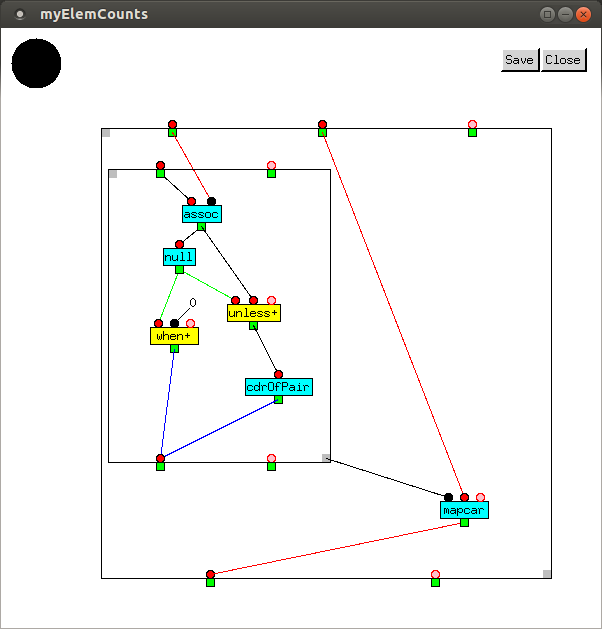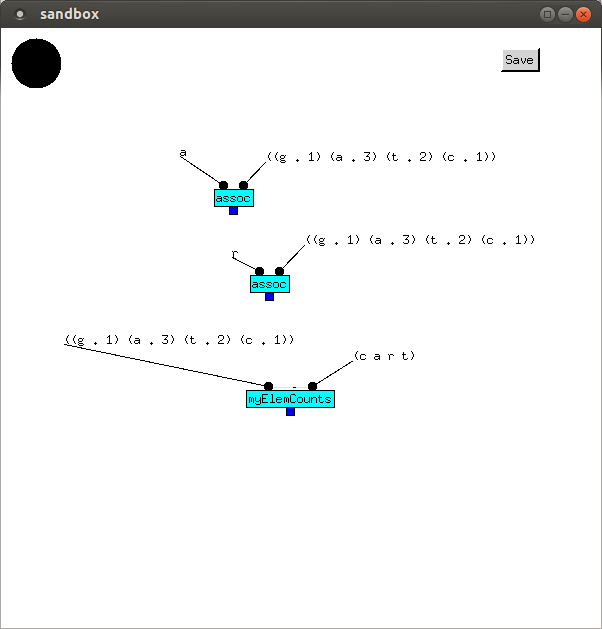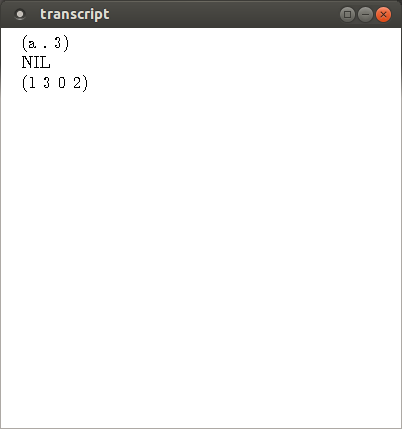# Enclosures and Sticky Values

In this example, we extract values from a `Bag`. A `Bag` is like a list, except that its elements are unordered, and like a set, except that it stores how many of each element it contains. For example, the `List` `(g a t t a c a)` contains 1 of `g`, 3 of `a`, 2 of `t` and 1 of `c`. As a set, it is `(g a t c)`, but the order of elements doesn't matter. As a `Bag`, it is `((g . 1) (a . 3) (t . 2) (c . 1))`. It preserves how many of each element it contains, but like the set, the order doesn't matter.

`myElemCounts` is an implementation of `elemCounts`, a function in Emblem's `Bag` library. It takes a `Bag` and a list of elements, and returns a list containing how many elements of each type contained in the list are present in the `Bag`.`myElemCounts` calls `mapcar`, which applies its first argument, a function - here an Enclosure - to each element in its remaining arguments. Here, there is one remaining argument, the list `(c a r t)`.

The enclosure calls the function `assoc`, whose first argument is a key, and returns the corresponding (key . value) pair in its second argument, or `NIL` if there is none.

 Inputs Vertex Outputs `c((g . 1) (a . 3) (t . 2) (c . 1))` `assoc` `(c . 1)` `(c . 1)` `null` `NIL` `NIL0` `when+` `NIL(c . 1)` `unless+` `(c . 1)` `(c . 1)` `cdrOfPair` `1`

 Inputs Vertex Outputs `a((g . 1) (a . 3) (t . 2) (c . 1))` `assoc` `(a . 3)` `(a . 3)` `null` `NIL` `NIL0` `when+` `NIL(a . 3)` `unless+` `(a . 3)` `(a . 3)` `cdrOfPair` `3`

 Inputs Vertex Outputs `r((g . 1) (a . 3) (t . 2) (c . 1))` `assoc` `NIL` `NIL` `null` `T` `T0` `when+` `0` `TNIL` `unless+` `cdrOfPair`

 Inputs Vertex Outputs `t((g . 1) (a . 3) (t . 2) (c . 1))` `assoc` `(t . 2)` `(t . 2)` `null` `NIL` `NIL0` `when+` `NIL(t . 2)` `unless+` `(t . 2)` `(t . 2)` `cdrOfPair` `2`

You might have noticed that although the first input to `assoc` changes, the second one doesn't. Four different values are supplied to the first input while `mapcar` is executing,but only one value is supplied to the second input, when `myElemCounts` is called. To prevent the second input value being consumed along with the first input value `c`, it has been made sticky, which is why, like constant values, it is shown as black.

`assoc` is called twice in the sandbox before `myElemCounts` is called.